##### Quantum Physics For Dummies, Revised EditionIn quantum physics, when working with kets, it is useful to know how to use eigenvectors and eigenvalues. Applying an operator to a ket can result in a new ket: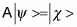To make things easier, you can work with eigenvectors and eigenvalues (eigen is German for “innate” or “natural”). For example,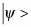is an eigenvector of the operator A if

• The number a is a complex constant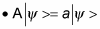Note what’s happening here: Applying A to one of its eigenvectors,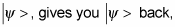multiplied by that eigenvector’s eigenvalue, a.

Although a can be a complex constant, the eigenvalues of Hermitian operators are real numbers, and their eigenvectors are orthogonal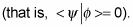Casting a problem in terms of eigenvectors and eigenvalues can make life a lot easier because applying the operator to its eigenvectors merely gives you the same eigenvector back again, multiplied by its eigenvalue — there’s no pesky change of state, so you don’t have to deal with a different state vector.

Take a look at this idea, using the R operator from rolling the dice, which is expressed this way in matrix form: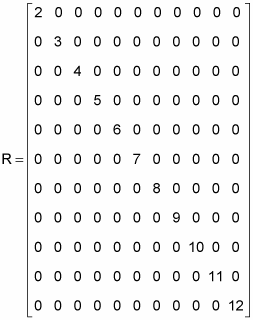The R operator works in 11-dimensional space and is Hermitian, so there’ll be 11 orthogonal eigenvectors and 11 corresponding eigenvalues.

Because R is a diagonal matrix, finding the eigenvectors is easy. You can take unit vectors in the 11 different directions as the eigenvectors. Here’s what the first eigenvector,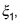would look like: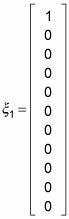And here’s what the second eigenvector,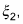would look like: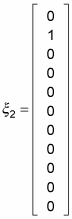And so on, up to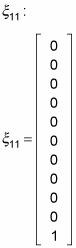Note that all the eigenvectors are orthogonal.

And the eigenvalues? They’re the numbers you get when you apply the R operator to an eigenvector. Because the eigenvectors are just unit vectors in all 11 dimensions, the eigenvalues are the numbers on the diagonal of the R matrix: 2, 3, 4, and so on, up to 12.